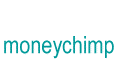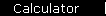How Finance Works   Most of finance is built from a few standard formulas and strategies. For example, investments tend to grow like compound interest and pay out like annuities; and you judge them by estimating future profits and then calculating present values or equivalent rates of return. So here are the basic building blocks, from the easy to the ugly. This article links to interactive calculators to demonstrate some key financial concepts; just using the calculators will be enough for most people. But if you're nuts about numbers, you can also see where the various formulas come from.   home  |  article  |  glossary  |  calculator  |  about us  |  booksIntroductionCompound InterestMore CompoundingPresent Value / CAGRComposite InvestmentsBond YieldGeometric SeriesGrowth + ContributionsAnnuityMortgageStock ValuationBooks & Links